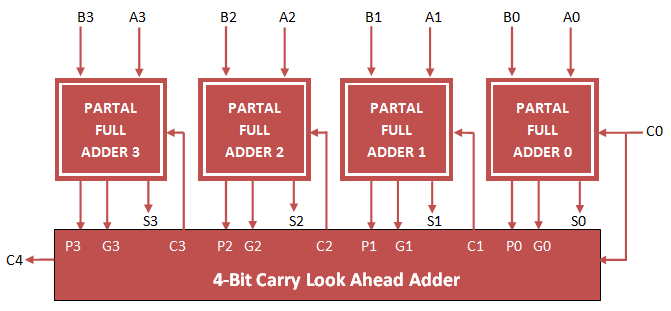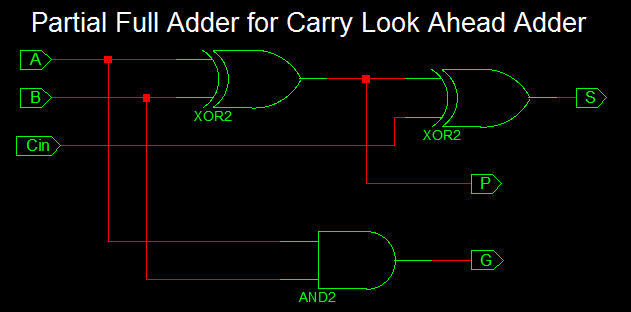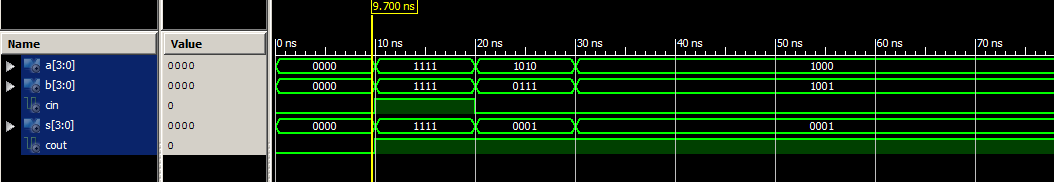In order to implement Carry Look Ahead Adder, first implement Partial Full Adder and then Carry logic using Propagation and generation Block.Partial Full Adder consist of inputs (A, B, Cin) and Outputs (S, P, G) where P is Propagate Output and G is Generate output.

VHDL code for carry look ahead adder can be implemented by first constructing Partial full adder block and port map them to four times and also implementing carry generation block as shown below.### VHDL Code for Partial Full Adder

```
library IEEE;
use IEEE.STD_LOGIC_1164.ALL;

Port ( A : in STD_LOGIC;
B : in STD_LOGIC;
Cin : in STD_LOGIC;
S : out STD_LOGIC;
P : out STD_LOGIC;
G : out STD_LOGIC);

begin

S <= A xor B xor Cin;
P <= A xor B;
G <= A and B;

end Behavioral;

```

```
library IEEE;
use IEEE.STD_LOGIC_1164.ALL;

Port ( A : in STD_LOGIC_VECTOR (3 downto 0);
B : in STD_LOGIC_VECTOR (3 downto 0);
Cin : in STD_LOGIC;
S : out STD_LOGIC_VECTOR (3 downto 0);
Cout : out STD_LOGIC);

Port ( A : in STD_LOGIC;
B : in STD_LOGIC;
Cin : in STD_LOGIC;
S : out STD_LOGIC;
P : out STD_LOGIC;
G : out STD_LOGIC);
end component;

signal c1,c2,c3: STD_LOGIC;
signal P,G: STD_LOGIC_VECTOR(3 downto 0);
begin

PFA1: Partial_Full_Adder port map( A(0), B(0), Cin, S(0), P(0), G(0));
PFA2: Partial_Full_Adder port map( A(1), B(1), c1, S(1), P(1), G(1));
PFA3: Partial_Full_Adder port map( A(2), B(2), c2, S(2), P(2), G(2));
PFA4: Partial_Full_Adder port map( A(3), B(3), c3, S(3), P(3), G(3));

c1 <= G(0) OR (P(0) AND Cin);
c2 <= G(1) OR (P(1) AND G(0)) OR (P(1) AND P(0) AND Cin);
c3 <= G(2) OR (P(2) AND G(1)) OR (P(2) AND P(1) AND G(0)) OR (P(2) AND P(1) AND P(0) AND Cin);
Cout <= G(3) OR (P(3) AND G(2)) OR (P(3) AND P(2) AND G(1)) OR (P(3) AND P(2) AND P(1) AND G(0)) OR (P(3) AND P(2) AND P(1) AND P(0) AND Cin);

end Behavioral;

```

```
LIBRARY ieee;
USE ieee.std_logic_1164.ALL;

-- Component Declaration for the Unit Under Test (UUT)

PORT(
A : IN std_logic_vector(3 downto 0);
B : IN std_logic_vector(3 downto 0);
Cin : IN std_logic;
S : OUT std_logic_vector(3 downto 0);
Cout : OUT std_logic
);
END COMPONENT;

--Inputs
signal A : std_logic_vector(3 downto 0) := (others => '0');
signal B : std_logic_vector(3 downto 0) := (others => '0');
signal Cin : std_logic := '0';

--Outputs
signal S : std_logic_vector(3 downto 0);
signal Cout : std_logic;

BEGIN

-- Instantiate the Unit Under Test (UUT)
A => A,
B => B,
Cin => Cin,
S => S,
Cout => Cout
);

-- Stimulus process
stim_proc: process
begin
-- hold reset state for 100 ns.
wait for 10 ns;

A <= "1111";
B <= "1111";
Cin <= '1';

wait for 10 ns;

A <= "1010";
B <= "0111";
Cin <= '0';

wait for 10 ns;

A <= "1000";
B <= "1001";
Cin <= '0';

wait;

end process;

END;

```1.2.